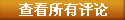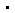﻿ 活动星系核致密射电源也会“眨眼”—论文—科学网
﻿

 活动星系核致密射电源也会“眨眼”

打印  发E-mail给：

﻿
2015/9/28 18:43:01 trafagar
2.广义基本力武器（人造作用力武器）【国家的机密】 ∑ε_ijk D_k(D_j(D_i(ψ×ψ^T)))=0 （D_1为人造动量场算子，D_2(ψ×ψ^T)=(e^(i λ_2(x_μ)*T_^a))*(ψ×ψ^T),D_3=e^(i λ_3(x_μ)*T_^a*(ψ×ψ^T))*(ψ×ψ^T)分别为对称变换）-->D_1(ψ×ψ^T)=f(ψ,A),D"_1(ψ×ψ^T)=f(ψ,A") -->∑ ε_ijk L(ψ,D_k(D_j(D_iψ)),A)=0 --> 广义鬼场η_1,η_2 -->黄淑懿规则(广义费曼规则)相关新闻 相关论文
﻿
 图片新闻>>更多

 一周新闻排行 一周新闻评论排行

 编辑部推荐博文 ﻿你跳了吗？科研中的拖延症指挥棒作用浅析：谈四轮学科评估指标体系科技写作的“魔鬼训练”药物研发新思路: 先药后病“文学与自然科学研究中心”研究什么？

 论坛推荐 ﻿矩阵分析 霍恩 (Roger A.Horn)著弦论的发展史波恩的光学原理现代数学手册AP版数理物理学百科 3324页时间与物理学